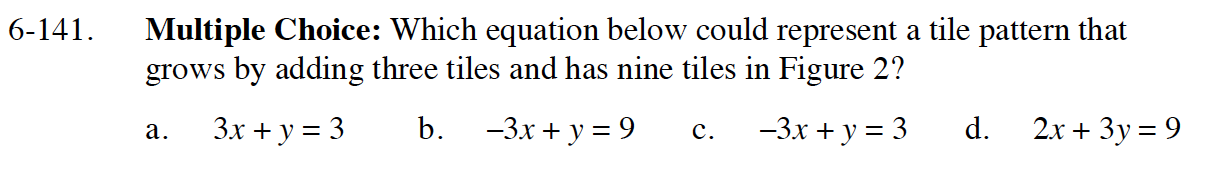Home > GB8I > Chapter cc46 > Lesson cc46.4.2 > Problem6-141

6-141.
1. Multiple Choice: Which equation below could represent a tile pattern that grows by adding three tiles and has nine tiles in Figure 2? Homework Help ✎

1. 3x + y = 3

2. −3x + y = 9

3. −3x + y = 3

4. 2x + 3y = 9Growth is the rate of change or the slope, in slope intercept form (y = mx + b) what variable represents the growth?

Knowing figure 2 has nine tiles, how can you use the growth to find the number of tiles in figure number 0 (starting figure)?

Write the equation in slope intercept form, then rearrange to Standard form (Ax + By = C).

(c)